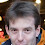## Wednesday, February 02, 2005

### How to Judge a Weatherman?

Each day a weatherman gives a probability p of rain for the next day and each day it either rains or it doesn't. How do we judge the quality of these forecasts? A first attempt uses linear scores, p if it rains, 1-p if it doesn't. However when you analyze this system the weatherman should predict p=1 if his belief is greater than 1/2 and p=0 otherwise.

A better measure is the log loss. The weatherman gets penalized -log(p) if it rains and -log(1-p) if it doesn't. A weatherman now has the incentive to announce his belief. There are other scoring functions with this property but the log loss has some nice properties such as the best a weather could hope to achieve is exactly the entropy of the distribution. The log loss and other measures are often used to analyze prediction mechanisms such as information markets.

Dean Foster and Rakesh Vohra have a different take looking at a notion called calibration. Here you take all the days that the weatherman predicted 70% chance of rain and check that 70% of those days it actually rained. A prediction algorithm calibrates a binary sequence if for finite set of allowed probabilities, each of the subsequences consisting of predictions of probability p have about a p fraction of ones. Foster and Vohra showed that some probabilistic calibration scheme will calibrate every sequence in the limit. In other words you can be a great weatherman in the calibration sense just by looking at the history of rain and forgoing that pesky meterological training.

Dean Foster and Sham Kakade gave a couple of interesting talks at the Bounded Rationality workshop giving a deterministic scheme that achieves a weak form of calibration and use it to learn Nash equilibirum in infinite repeated games.

1.Back when the Usenet was popular, a recurring question was "what do the weatherman probabilities mean?". This was well over a decade ago but I seem to recall that the answer was: the weather forecast service runs a few different computer models (usually 5 of them) and sees what is the outcome 24 hours into the future. If two models predict rain, then the probability is 40%, if 4 of them predict rain then it is 80%.

At the risk of restarting a long dead usenet thread, can any one out there confirm this?

2.This is old, but may shed some light on the issue:
http://www.straightdope.com/classics/a2_115b.html

3.It seems that both are correct.

Some weatherforecasters use statistical data to search for other times where weather conditions were the same as today. In this case the probability is historical: in 20% of the days that were just like today it ended up raining.

Others run a set of computer models or the same computer model with small variations (known as ensemble) and report the percentage of such outcomes. It rained in 20% of our computer simulations. Each outcome can be weighted to reflect the probability of a given variation.

http://www.metoffice.com/research/nwp/publications/nwp_gazette/dec99/predict.html

http://www.weatherzone.com.au/misc/glossary.jsp?letter=P
For instance, if the chance of receiving above-median rainfall in a particular climate scenario is 60%, then 60% of past years when that scenario occurred had above median rainfall, and 40% had below-median rainfall.

http://www.ucar.edu/research/tools/models.shtml
Because of weather's chaotic nature, errors or uncertainties in the starting point of a model can alter the results dramatically. One way to reduce the impact of such errors is through an ensemble of forecasts. In this technique, one model is run several times, each with a slightly different, intentionally varied set of starting points.

4.I once thought about this same question, in the context of how to get students to reveal their probabilities on true-or-false tests. My solution was to give p^2 points for each question if the answer is "true," or (1-p)^2 points if the answer is false. Lance, do you know what the properties of the log-loss function are that make it preferable?

5.Duhhh... I meant penalize by p^2 points if the answer is false, or by (1-p)^2 if the answer is true.

6.One nice things about logs is that uncertainty becomes additive. For instance if your students were completely ignorant, and you replaced your 10 binary questions with 1 question having 1024 answers, they would still get the same number of points if using log p award scheme.

Using log(p) as length for codeword of symbol with probability p is also guaranteed to produce lowest expected codeword length when codewords must be prefix-free (instantaneously decodable). I wonder, what sort of bounds would hold for codes without any such constraints?

Finally, using f(x)=log x as a way to award points would elicit correct internal probabilities from rational students, whereas f(x)=x^2 will not. This seemed like an interesting topic, hence my first blog entry has a derivation of this :)
http://yaroslavvb.blogspot.com/